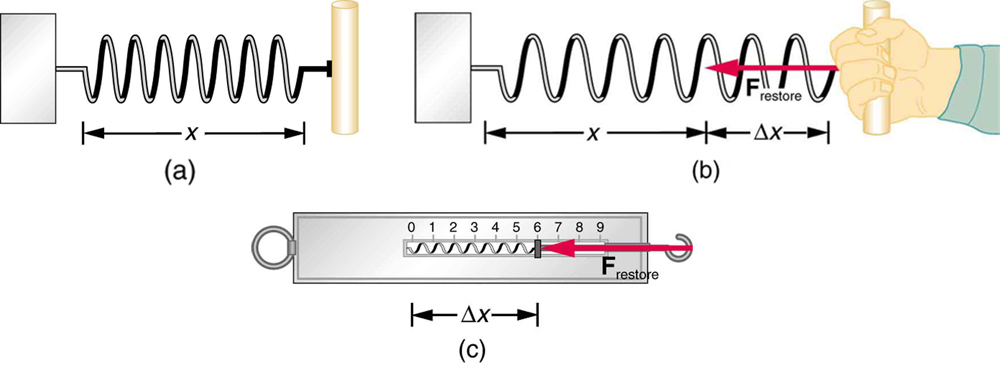# If a spring has a constant of 8 (kg)/s^2, how much work will it take to extend the spring by 40cm ?

Mar 18, 2018Recall Hooke's Law,

${F}_{\text{s}} = - k x$, and by extension

${F}_{\text{ext" = -F_"s}} = k x$ (we're looking to find this)

where $k$ is the constant you describe and $x$ is the displacement of spring.

Moreover, recall that work is represented by,

$W = F \cdot d$

Now,

$\implies W = \left(\frac{1}{2} k x\right) \cdot x = \frac{1}{2} k {x}^{2}$

(the reason this is halved is because we're averaging the force)

Hence,

${W}_{\text{ext" = 1/2 * (8"kg")/("s"^2) * (0.40"m")^2 approx 0.64"J}}$

To be sure, the elastic potential energy is equal to the work done to stretch it that far (which shares the same equation as that derived).